Name:    Chapter B Test

Multiple Choice
Identify the choice that best completes the statement or answers the question.

Tell which number is greater.

1.

–6, 13
 a. –13 c. –6 b. 6 d. 13

Simplify the expression.

2.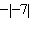a. 7 c. –7 b.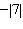d.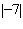Use a number line to find the sum or difference.

3.

–7 – 5
 a. 2 c. –12 b. 12 d. –2

4.

–15 – 5
 a. 20 c. 10 b. –20 d. –10

5.

0 – 8
 a. 80 c. 8 b. –80 d. –8

Use a number line to evaluate the expression.

6.

–2 + 9 + 7
 a. 0 c. –4 b. –14 d. 14

7.

7 – 8 – 2
 a. –17 c. 1 b. –3 d. 13

Find the median of the data.

8.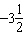,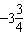,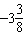,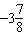,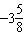a.c.b.d.9.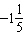,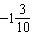,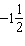,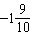,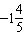a.c.b.d.Use a number line to find the sum or difference.

10.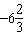+a.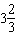c.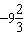b.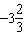d.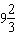11.

How many quadrants are in a coordinate plane?
 a. 3 c. 2 b. 1 d. 4

Write the ordered pair that corresponds to the point.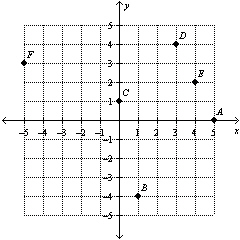12.

Point C
 a. (0, –1) c. (1, 0) b. (–1, 0) d. (0, 1)

Numeric Response

Find the absolute value of the integer.

1.

–14

Use a number line to find the sum or difference.

2.

0 + 6.3

Which number is greater? Explain.

1.

–8.2, –8.02

2.

Without using a number line, determine if the sum of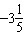and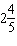is positive or negative. Explain.

3.

Two vertices of triangle ABC are A(–3, –3) and B(7, –3). List four possible coordinates of the third vertex so that the triangle has an area of 25 square units.